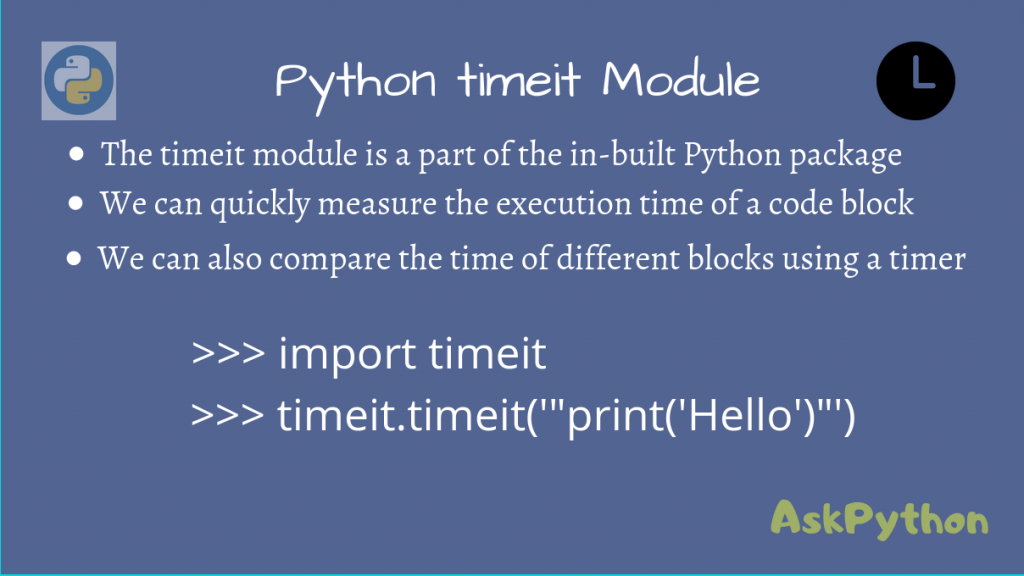# Python timeit ModuleThe Python timeit module is a simple interface to quickly measure the execution time for small blocks of code.

When you are creating an application, you may wonder how this block of code will perform and would want to test it under different scenarios.

For this, the `timeit` module provides a very simple solution to this problem. Let’s look at how we can use this to time our code snippets!

We shall be looking at both the Command Line Interface and a Callable interface.

## Python timeit – Command Line Interface

The command-line interface is very similar to that of running a Python program.

You need to import the external module `timeit` using the -m option and apply it to your code.

```python -m timeit 'print("Hello from AskPython")'
```

This will run the snippet, passed as a string, using `timeit`.

By default, this will run the code 1 million times on Linux and 20 million times on Windows, and measure the best time among those values. The below outputs are from my Linux system.

Note that if you already have a for loop in your snippet, the module will ensure that the total number of iterations is close to 1 million, so your entire loop will not run for 1 million times!

## Python timeit – Python Interface

We can also use `timeit` via the Python interpreter, and import it using:

```import timeit
```

To find the execution time, pass the code as a string to `timeit.timeit()`.

```execution_time = timeit.timeit(code, number)
```

We can control the number of iterations using the `number` parameter.

```>>> import timeit
>>> timeit.timeit('"-".join(str(n) for n in range(100))', number=10000)
0.19053685299877543
>>> timeit.timeit('"-".join([str(n) for n in range(100)])', number=10000)
0.172546762998536
>>> timeit.timeit('"-".join(map(str, range(100)))', number=10000)
0.13625987299747067
>>>
```

## Using the timeit Module

Let’s now look at how we can use `timeit` to time a snippet, inside our program.

But before that, you may be wondering about something. What if your code required some previous setup to be done? And if you need to import certain modules too?

Well, the solution to this problem is to use a setup code block, which will do all the necessary work in setting up all required modules and variables.

```setup_code = "import math"
```

Writing a setup block is very simple. You simply write whatever code you need, and pass it as a string, into a variable.

After this, you can write your main code block and pass it to `timeit.timeit()`, using the `setup` and `stmt` parameters.

```execution_time = timeit.timeit(setup = setup_code, stmt = main_block, number = 100)
```

`timeit` will ensure that the setup is done before your main loop is measured, so it is only executed once!

Let’s quickly look at an example now.

This code tries to get all sub-arrays from the starting element of a numpy array. Notice that the setup block is run only once.

```import timeit

# Setup is run only once
setup_code = '''
import numpy as np
a = np.arange(0, 1000)
print(a.shape)
def print_subarrays(a):
op = []
for i in range(a.shape):
op.append(a[:i])
'''

main_block = '''
print_subarrays(a)
'''

# Main Block is run 1000 times
print('Best execution Time among 1000 iterations:', timeit.timeit(setup=setup_code, stmt=main_block, number=1000))

```

Output

```(1000,)
Best execution Time among 1000 iterations: 0.3830194959991786
```

## Compare the Performance of Code Blocks

We can easily compare the performance of multiple blocks of code using `timeit`.

We will use a timer for this purpose, using `timeit.default_timer()`.

The time taken for a code block will be the current time minus the initial time taken as reference, which you can pass through variables.

```import timeit

start_time = timeit.default_timer()
function_1()
time_1 = timeit.default_timer() - start_time

start_time = timeit.default_timer()
function_2()
time_2 = timeit.default_timer() - start_time

print('Function 1 took', time_1)
print('Function 2 took', time_2)
```

Let’s test 2 functions on a numpy array `range()` and `np.arange()` and see how they compare.

```import timeit
import numpy as np

def time_range(size):
for i in range(size):
pass

def time_arange(size):
np.arange(size)

if __name__ == '__main__':
# For smaller arrays
print('Array size: 1000')

start_time = timeit.default_timer();
time_range(1000)
range_time_1 = timeit.default_timer() - start_time

start_time = timeit.default_timer();
time_arange(1000)
arange_time_1 = timeit.default_timer() - start_time

# For large arrays
print('Array size: 1000000')

start_time = timeit.default_timer();
time_range(1000000)
range_time_2 = timeit.default_timer() - start_time

start_time = timeit.default_timer();
time_arange(1000000)
arange_time_2 = timeit.default_timer() - start_time

print(f'size 1000: range() took {range_time_1}')
print(f'size 1000: arange() took {arange_time_1}')
print(f'size 1000000: range() took {range_time_2}')
print(f'size 1000000: arange() took {arange_time_2}')

```

Output

```Array size: 1000
Array size: 1000000
size 1000: range() took 2.2970001737121493e-05
size 1000: arange() took 8.393999451072887e-06
size 1000000: range() took 0.02567379199899733
size 1000000: arange() took 0.0031752489994687494
```

Thus, we could easily use `timeit` to compare the performance of different functions.

## Time a Particular Function

We can also only time the performance of a specific function in a script, without running the other code blocks.

If the earlier file was called numpy_compare.py, we can find the execution time using the below invocation:

```python -m timeit -s 'import numpy_compare' 'numpy_compare.time_range(1000)'
```

Output